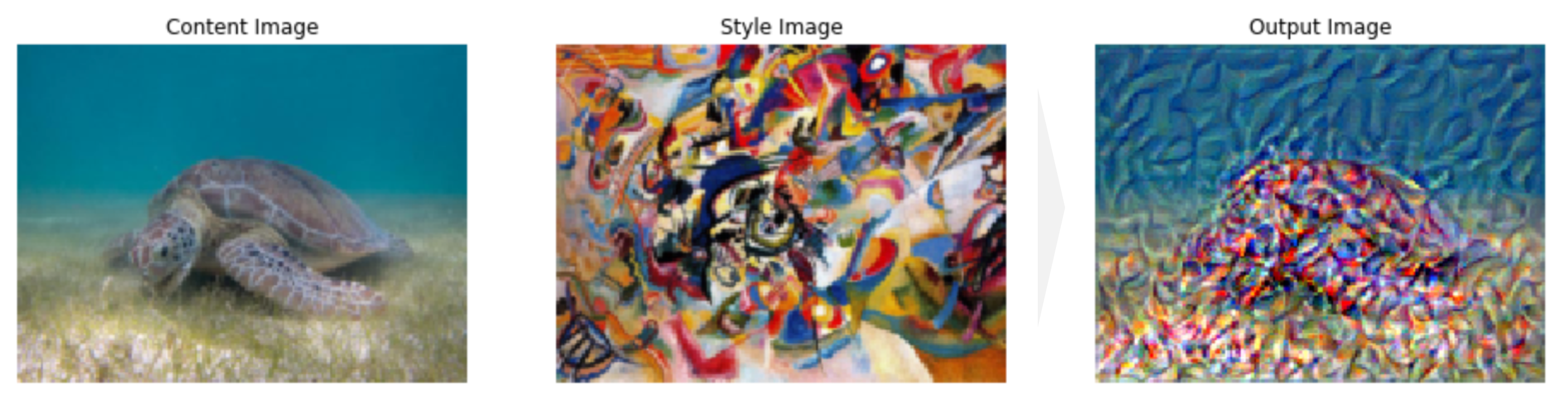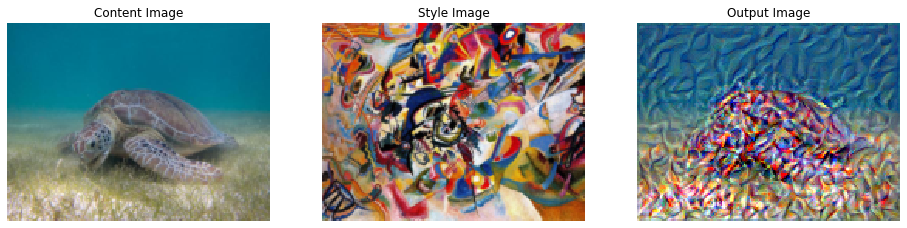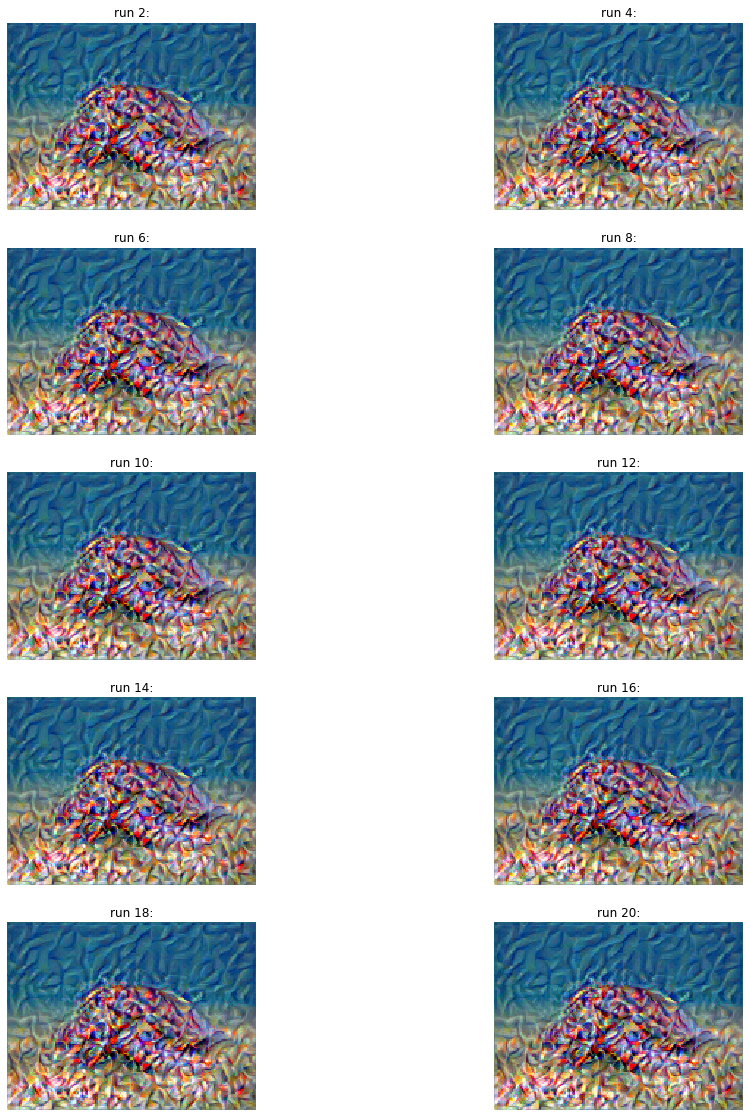# Style Transfer using Pytorch (Part 4)

## Goal¶

This post aims to explain the concept of style transfer step-by-step. Part 4 is about executing the neural transfer.Reference

## Libraries¶

In :
import pandas as pd
import copy

# Torch & Tensorflow
import torch
import torch.nn as nn
import torch.nn.functional as F
import torchvision
import tensorflow as tf

# Visualization
from torchviz import make_dot
from PIL import Image
import matplotlib.pyplot as plt
%matplotlib inline

import warnings


## Configuration¶

In :
device = torch.device("cuda" if torch.cuda.is_available() else "cpu")


## Functions¶

The functions covered by the previous posts (Part 1, Part 2, Part 3) are as follows.

### Functions from Part 1 - image loader¶

In :
# desired size of the output image
imsize = (512, 512) if torch.cuda.is_available() else (128, 128)  # use small size if no gpu

torchvision.transforms.Resize(imsize),  # scale imported image
torchvision.transforms.ToTensor()])  # transform it into a torch tensor

image = Image.open(image_name)

# fake batch dimension required to fit network's input dimensions
return image.to(device, torch.float)

In :
unloader = torchvision.transforms.ToPILImage()

def imshow_tensor(tensor, ax=None):
image = tensor.cpu().clone()  # we clone the tensor to not do changes on it
image = image.squeeze(0)      # remove the fake batch dimension

if ax:
ax.imshow(image)
else:
plt.imshow(image)


### Functions from Part 2 - loss functions¶

In :
class ContentLoss(nn.Module):

def __init__(self, target,):
super(ContentLoss, self).__init__()
self.target = target.detach()

def forward(self, input):
self.loss = F.mse_loss(input, self.target)
return input

def gram_matrix(input):
# Get the size of tensor
# a: batch size
# b: number of feature maps
# c, d: the dimension of a feature map
a, b, c, d = input.size()

# Reshape the feature
features = input.view(a * b, c * d)

# Multiplication
G = torch.mm(features, features.t())

# Normalize
G_norm = G.div(a * b * c * d)
return G_norm

class StyleLoss(nn.Module):

def __init__(self, target_feature):
super(StyleLoss, self).__init__()
self.target = gram_matrix(target_feature).detach()

def forward(self, input):
G = gram_matrix(input)
self.loss = F.mse_loss(G, self.target)
return input


### Functions from Part 3 - modeling¶

#### Normalization¶

In :
cnn_normalization_mean = torch.tensor([0.485, 0.456, 0.406]).to(device)
cnn_normalization_std = torch.tensor([0.229, 0.224, 0.225]).to(device)

# create a module to normalize input image so we can easily put it in a
# nn.Sequential
class Normalization(nn.Module):
def __init__(self, mean, std):
super(Normalization, self).__init__()
# .view the mean and std to make them [C x 1 x 1] so that they can
# directly work with image Tensor of shape [B x C x H x W].
# B is batch size. C is number of channels. H is height and W is width.
self.mean = torch.tensor(mean).view(-1, 1, 1)
self.std = torch.tensor(std).view(-1, 1, 1)

def forward(self, img):
# normalize img
return (img - self.mean) / self.std


#### Create a sequential model for style transfer¶

In :
# desired depth layers to compute style/content losses :
content_layers_default = ['conv_4']
style_layers_default = ['conv_1', 'conv_2', 'conv_3', 'conv_4', 'conv_5']

def get_style_model_and_losses(cnn, normalization_mean, normalization_std,
style_img, content_img,
content_layers=content_layers_default,
style_layers=style_layers_default):
cnn = copy.deepcopy(cnn)

# normalization module
normalization = Normalization(normalization_mean, normalization_std).to(device)

# just in order to have an iterable access to or list of content/syle
# losses
content_losses = []
style_losses = []

# assuming that cnn is a nn.Sequential, so we make a new nn.Sequential
# to put in modules that are supposed to be activated sequentially
model = nn.Sequential(normalization)

i = 0  # increment every time we see a conv
for n_child, layer in enumerate(cnn.children()):
#         print()
#         print(f"n_child: {n_child}")
if isinstance(layer, nn.Conv2d):
i += 1
name = 'conv_{}'.format(i)
elif isinstance(layer, nn.ReLU):
name = 'relu_{}'.format(i)
# The in-place version doesn't play very nicely with the ContentLoss
# and StyleLoss we insert below. So we replace with out-of-place
# ones here.
layer = nn.ReLU(inplace=False)
elif isinstance(layer, nn.MaxPool2d):
name = 'pool_{}'.format(i)
elif isinstance(layer, nn.BatchNorm2d):
name = 'bn_{}'.format(i)
else:
raise RuntimeError('Unrecognized layer: {}'.format(layer.__class__.__name__))

#         print(f'Name: {name}')
if name in content_layers:
target = model(content_img).detach()
content_loss = ContentLoss(target)
content_losses.append(content_loss)

if name in style_layers:
target_feature = model(style_img).detach()
style_loss = StyleLoss(target_feature)
style_losses.append(style_loss)

# now we trim off the layers after the last content and style losses
for i in range(len(model) - 1, -1, -1):
if isinstance(model[i], ContentLoss) or isinstance(model[i], StyleLoss):
break

model = model[:(i + 1)]

return model, style_losses, content_losses


In :
d_path = {}

In :
style_img = image_loader(d_path['style'])[:, :, :, :170]
content_img = image_loader(d_path['content'])[:, :, :, :170]
input_img = content_img.clone()

assert style_img.size() == content_img.size(), \
"we need to import style and content images of the same size"


## Modeling¶

In :
# Obtain the model for style transfer
# with warnings.catch_warnings():
warnings.filterwarnings("ignore")
cnn = torchvision.models.vgg19(pretrained=True).features.to(device).eval()
model, style_losses, content_losses = get_style_model_and_losses(cnn, cnn_normalization_mean, cnn_normalization_std, style_img, content_img)


## Executing a neural transfer¶

L-BFGS stands for Limited-memory Broyden–Fletcher–Goldfarb–Shanno according to wiki - Limited-memory_BFGS, which is one of the optimization algorithm using limited amount of memory.

In :
def get_input_optimizer(input_img):
# this line to show that input is a parameter that requires a gradient
return optimizer

optimizer = get_input_optimizer(input_img)


### Execution¶

The execution steps in the function get_style_model_and_losses in NEURAL TRANSFER USING PYTORCH are as follows:

1. Initialization
2. Parameter
3. Define closure function to re-evaluate the model to execute the followings:
• masking images between 0 and 1 by .clamp method
• reset gradient by zero_grad method
• reset the error score for style and content
• compute the style and content loss in each inserted layer
• compute the sum of the losses for style and content
• multiply the weight for style and content to manipulate the style transfer balance by input argument
• execute error back propagation
4. Execute the steps by gradient descent optimizer
In :
# Parameters
num_steps = 10
style_weight=5000
content_weight=1
input_img = content_img[:, :, :, :170].clone()
d_images = {}

print('Building the style transfer model..')
model, style_losses, content_losses = get_style_model_and_losses(cnn,
cnn_normalization_mean, cnn_normalization_std, style_img, content_img)
optimizer = get_input_optimizer(input_img)

# Execution
run = 
while run <= num_steps:

def closure():
# correct the values of updated input image
input_img.data.clamp_(0, 1)

model(input_img)
style_score = 0
content_score = 0

for sl in style_losses:
style_score += sl.loss
for cl in content_losses:
content_score += cl.loss

style_score *= style_weight
content_score *= content_weight

loss = style_score + content_score
loss.backward()

run += 1
if run % 2 == 0:
print("run {}:".format(run))
print('Style Loss : {:4f} Content Loss: {:4f}'.format(
style_score.item(), content_score.item()))
input_img.data.clamp_(0, 1)
d_images[run] = input_img
print()

return style_score + content_score

optimizer.step(closure)

# a last correction...
input_img.data.clamp_(0, 1)

Building the style transfer model..
run :
Style Loss : 1004.939392 Content Loss: 0.000014

run :
Style Loss : 644.263489 Content Loss: 24.647982

run :
Style Loss : 558.792542 Content Loss: 55.995193

run :
Style Loss : 241.166168 Content Loss: 41.970711

run :
Style Loss : 143.137131 Content Loss: 51.402943

run :
Style Loss : 88.965408 Content Loss: 55.758999

run :
Style Loss : 57.654659 Content Loss: 60.926662

run :
Style Loss : 48.282879 Content Loss: 57.995407

run :
Style Loss : 36.090813 Content Loss: 58.100056

run :
Style Loss : 26.983953 Content Loss: 56.346275


In :
fig, axes = plt.subplots(1, 3, figsize=(16, 8))
d_img = {"Content": content_img,
"Style": style_img,
"Output": input_img}
for i, key in enumerate(d_img.keys()):
imshow_tensor(d_img[key], ax=axes[i])
axes[i].set_title(f"{key} Image")
axes[i].axis('off')### Visualize the process of style transfer¶

This is not yet obvious to see the processes of style transfer. It seems run 2 already finish most of the transfer processes. This needs to be investigated later.

In :
fig, axes = plt.subplots(int(len(d_images)/2), 2, figsize=(16, 20))
for i, key in enumerate(d_images.keys()):
imshow_tensor(d_images[key], ax=axes[i//2][i%2])
axes[i//2][i%2].set_title("run {}:".format(key))
axes[i//2][i%2].axis('off')